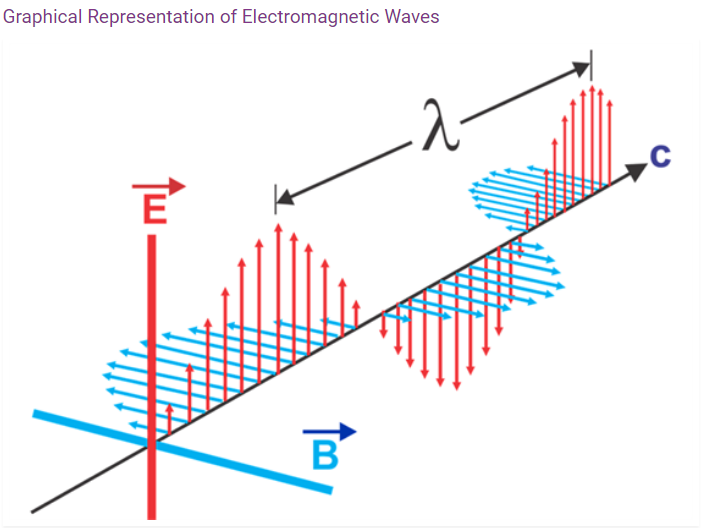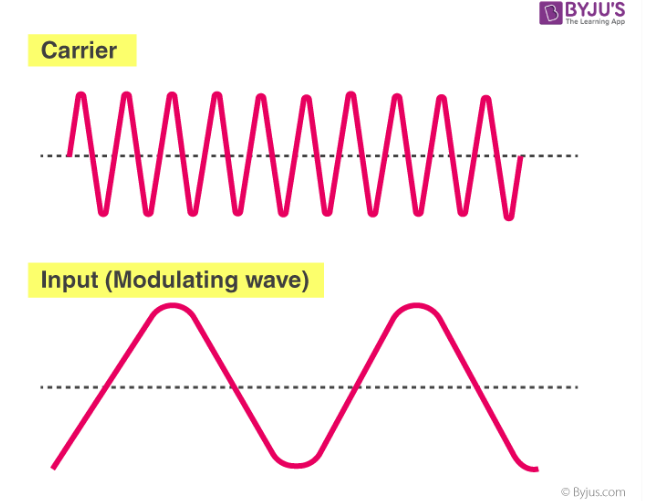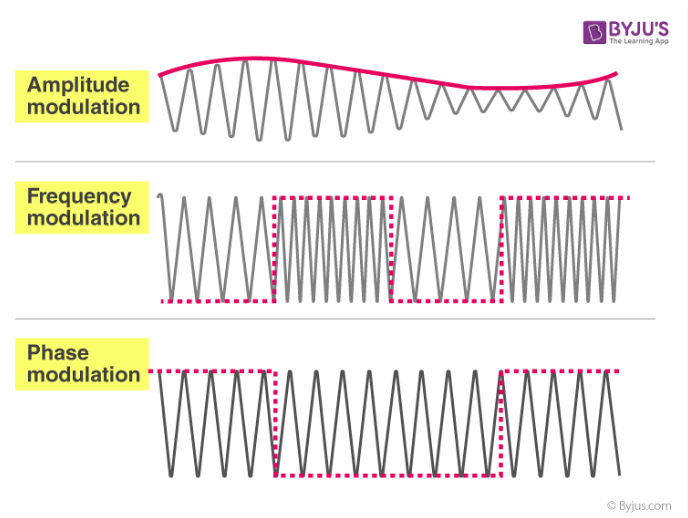Learn about smartphone sensors in the next Xcel masterclass with Tinkerbee CEO, Anupam! Learn about smartphone sensors in the next Xcel masterclass with Tinkerbee CEO, Anupam!

# RBSE Solutions For Class 12 Physics Chapter 17: Electromagnetic Waves Communication and Contemporary Physics | Textbook Important Questions & Answers

The answers are provided for all the questions of Chapter 17 Physics of RBSE Class 12. Students can go through these questions to understand the concepts better and score well in the board examination and entrance examinations for various professional courses. These solutions are provided by the team of experts and offer the best and accurate solutions to the questions. Do check BYJU’S RBSE Class 12 solutions page to get the answers for textbook questions of all subjects.

## Multiple Choice Questions

Q1: Average energy density radiated in an electromagnetic wave is related to:

1. Only electric field
2. Only the magnetic field
3. Both electric and magnetic field
4. Average energy density is zero

Q2: Waves related to telecommunication are

1. Infrared
2. Visible light
3. Microwaves
4. Ultraviolet ray

Q3: Electromagnetic waves do not transport

1. Energy
2. Charge
3. Momentum
4. Information

Q4: If and are electric and magnetic field vectors of electromagnetic waves, then the propagation of electromagnetic is along

1. x
2. .

Q5: Which radiation has the least wavelength?

1. X-ray
2. Gamma-ray
3. Beta ray
4. Alpha ray

## Very Short Answer Type Questions

Q1: What is the speed of electromagnetic waves in a vacuum?

Answer: The speed of electromagnetic wave c = 3 X 108 m/s

Q2: For electromagnetic waves, what is the effect on the refractive index of Ionosphere on increasing height from Earth’s surface?

Answer: The refractive index decreases.

Q3: The vibration of electromagnetic wave propagation in X-direction is parallel to Y-axis. Then in which axis vibration of is parallel?

Answer: is parallel in Z-axis

Q4: For long-distance propagation, which method is used for propagation?

Answer: The method used for long-distance propagation is skywave propagation.

Q5: What are the frequency limits of sky waves in sending the signal to remote places?

Answer: The frequency limit is from 1.5 MHz to 30MHz.

Q6: Name the part of the communication system which converts the signal to suitable form to send on a communication channel to a receiver.

Answer: The transmitter converts the signal to a suitable form to send on a communication channel to a receiver.

Q7: Name the method used to superimpose signal on carrier wave>

Answer: Modulation is the method used to superimpose signals on a carrier wave.

Q8: What types of matter are studied in nanotechnology?

Answer: The matter below the size of 100 nm.

## Short Answer Type Questions

Q1: Name the components of the electromagnetic spectrum in decreasing order of wavelength.

Answer: The electromagnetic spectrum is generally divided into seven regions, in the order of decreasing wavelength

1. Radiowaves (103 m)
2. Microwaves (10-2 m)
3. Infrared rays (10-5 m)
4. Visible light (0.5 x 10-6 m)
5. Ultraviolet rays (∼ 10-8 m)
6. X-rays (∼ 10-10 m)
7. Gamma rays (∼ 10-12 m)

Q2: Write four main characteristics of electromagnetic waves.

1. Electromagnetic waves are propagated by oscillating perpendicular to each other.
2. They travel with a constant speed of 3 x10-8 m/s in a vacuum.
3. These ways are not deflected by electric or magnetic fields.
4. Electromagnetic waves can reflect or refract like light waves.

Q3: Explain ground waves and sky waves.

Answer: The radio signal emitted from any place on the earth can be received at any location by two possible ways

1. If the wave travels along the surface of the earth from one point to another is called a ground wave.
2. If the wave is directed towards the sky and after reflection from the ionosphere of Earth’s atmosphere reaches the desired location, the earth is called the skywave.

Q4: What is the communication system?

Answer: A communication system is a method of transferring information from one source to another. The messages are converted into electrical signals and then transmitted. The basic components of the communication system are the Transmitter, Carrier and Receiver.

Q5: Name the part of the communication system.

Answer: The basic components of the communication system are the Transmitter, Channel and Receiver.

Q6: Explain modulation.

Answer: Modulation is the process of adding a carrier wave to the signal that has the data to be transmitted.

Q7: Give the name of the instruments used to observe nanostructures.

Answer: Few instruments used to observe the nanostructures are Optical microscope, Electron microscope, Scanning probe microscope and Atomic force microscope.

## Essay Type Questions

Q1: Describe the various components of the electromagnetic wave and explain their characteristics.

The two components of the electromagnetic wave are the electric field and magnetic field. The electric field is formed due to the flow of voltage and the magnetic field from the flow of current.Characteristics of Electromagnetic Wave

1. Electromagnetic waves are transverse in nature. The electric and magnetic field are perpendicular to each other.
2. Accelerated charges produce electromagnetic waves.
3. These waves travel at a constant velocity of 3 x 108 m/s in the vacuum.
4. Electromagnetic waves do not require a medium to travel.
5. The frequency of the wave remains unchanged but wavelength changes when it travels from one medium to another.
6. It follows the law of superposition.
7. The oscillating electric and magnetic fields are in phase and their magnitudes have a constant ratio.
8. The electric energy is equal to the magnetic energy in electromagnetic waves.
9. The Poynting vector represents the energy transferred by electromagnetic waves per unit area.

Q2: Explain with diagram amplitude modulation, frequency modulation and phase modulation.Amplitude Modulation

The modulated wave carries the signal as the change in amplitude which can be sensed at the receiving end. The frequency and phase of the signal remain constant.

Frequency modulation

The amplitude and phase of the wave are unchanged. The frequency of the wave is changed as per the signal. When the signal amplitude is high the frequency is high and vice-versa.

Phase modulation

The Phase modulation is similar to frequency modulation. In both frequency modulation and phase modulation, there is a change frequency but in phase modulation, the wave either leads or lags as per the signal voltage

Q3: Describe some examples of nanostructures observed in nature.